浅谈算法——splay

BST（二叉查找树）是个有意思的东西，种类巨TM多，然后我们今天不讲其他的，我们今天就讲splay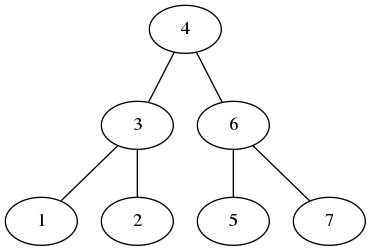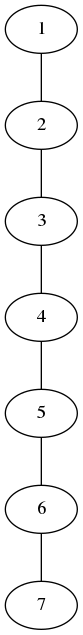1.旋转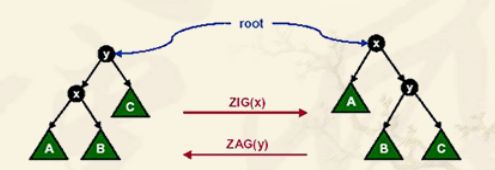ZIG和ZAG的结合也有几种情况#define ls(x) tree[x]
#define rs(x) tree[x]
#define T(x) (rs(f[x])==x)
void move(int x){
int fa=f[x],son=tree[x][T(x)^1];
tree[x][T(x)^1]=fa;
tree[fa][T(x)]=son;
if (son)	f[son]=fa;
f[x]=f[fa];
if (f[x])   tree[f[x]][T(fa)]=x;
f[fa]=x;
}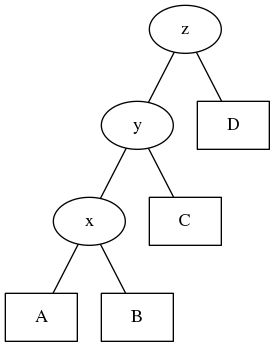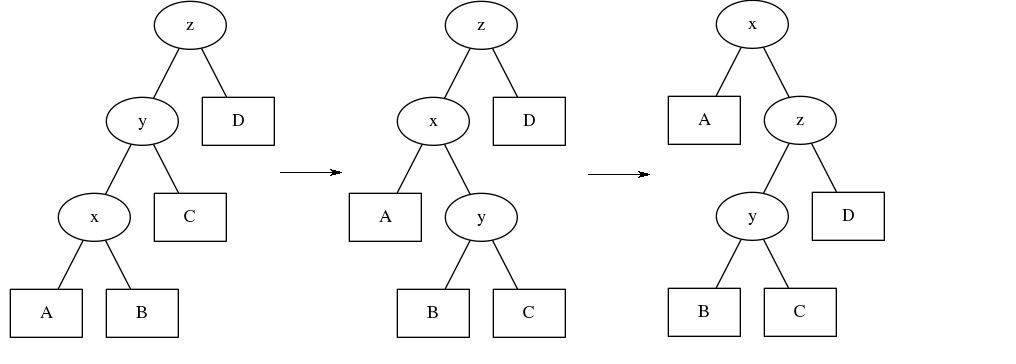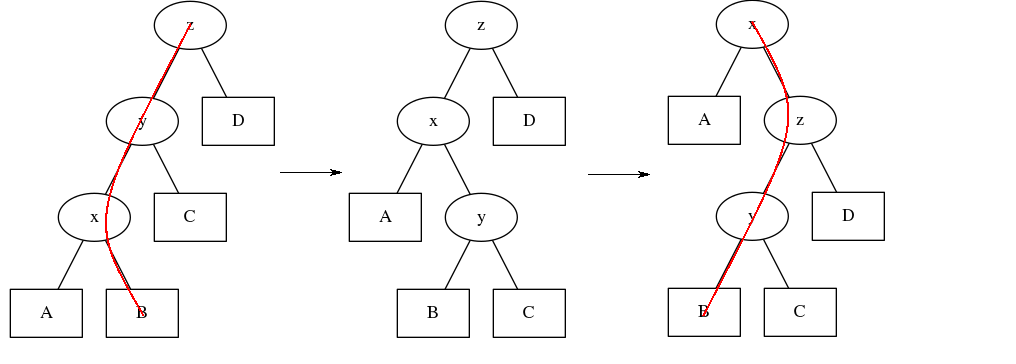void splay(int x){
while (f[x]){
if (f[f[x]])	T(x)==T(f[x])?move(f[x]):move(x);
move(x);
}
root=x;
}

Attention:move函数中的#define，对于之后的函数一直有效

2.插入

void insert(int x){
val[++len]=x;
if (!root){
size[root=len]=1;
return;
}
int i=root;
while (true){
size[i]++;
if (x<=val[i]){
if (!ls(i)){f[ls(i)=len]=i;break;}
i=ls(i);
}else{
if (!rs(i)){f[rs(i)=len]=i;break;}
i=rs(i);
}
}
splay(len);
}

3.找前驱/后继

int get_pre(){
int x=ls(root);
while (rs(x))   x=rs(x);
return x;
}
int get_suc(){
int x=rs(root);
while (ls(x))   x=ls(x);
return x;
}

4.查询

int find(int x,int i){
if (!i) return 0;
if (size[ls(i)]+1==x)   return i;
if (x<=size[ls(i)])  return find(x,ls(i));
return find(x-size[ls(i)]-1,rs(i));
}

5.删除

void Delete(int x){
splay(x);
if (!(ls(x)&&rs(x))){
f[root=ls(x)+rs(x)]=0;
clear(x);
return;
}
int i=get_pre();
splay(i);
size[f[rs(i)=rs(x)]=i]--;
clear(x);
}

6.区间操作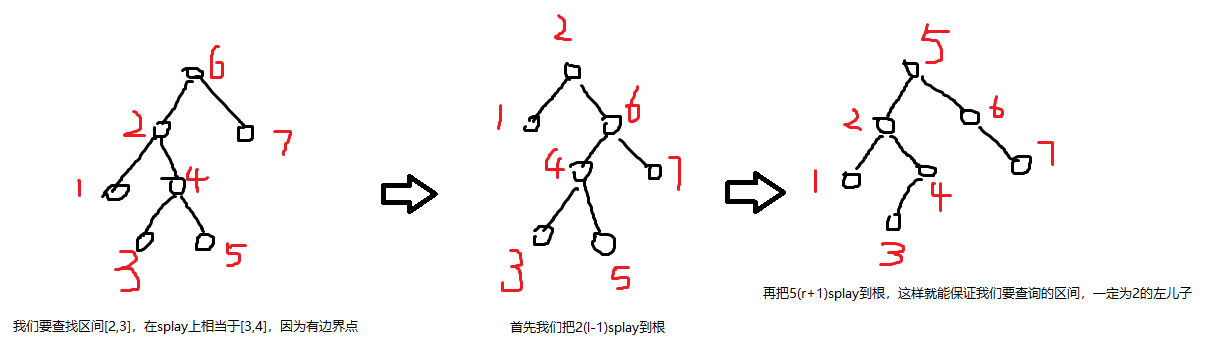splay的基本操作也就这些，下面我们来讲讲一个例题

例题

Tyvj 1728 普通平衡树
Description

1. 插入x数
2. 删除x数(若有多个相同的数，因只删除一个)
3. 查询x数的排名(若有多个相同的数，因输出最小的排名)
4. 查询排名为x的数
5. 求x的前驱(前驱定义为小于x，且最大的数)
6. 求x的后继(后继定义为大于x，且最小的数)

Input

Output

Sample Input
10
1 106465
4 1
1 317721
1 460929
1 644985
1 84185
1 89851
6 81968
1 492737
5 493598

Sample Output
106465
84185
492737

HINT
1.n的数据范围：n<=100000
2.每个数的数据范围：[-2e9,2e9]

splay经典板子题，用到之前说的所有操作。删点的话，先找排名，然后找点删除。至于查询排名，由于BST的优美性质，所以判断一下往左右递归即可

/*program from Wolfycz*/
#include<cmath>
#include<cstdio>
#include<cstring>
#include<iostream>
#include<algorithm>
using namespace std;
typedef long long ll;
typedef unsigned int ui;
typedef unsigned long long ull;
int x=0,f=1;char ch=getchar();
for (;ch<'0'||ch>'9';ch=getchar())	if (ch=='-')	f=-1;
for (;ch>='0'&&ch<='9';ch=getchar())  x=(x<<3)+(x<<1)+ch-'0';
return x*f;
}
inline void write(int x){
if (x>=10)   write(x/10);
putchar(x%10+'0');
}
const int N=1e5;
struct Splay{
#define ls(x) tree[x]
#define rs(x) tree[x]
#define T(x) (rs(f[x])==x)
int tree[N+10],f[N+10],size[N+10],val[N+10],root,len;
void updata(int x){size[x]=size[ls(x)]+size[rs(x)]+1;}
void clear(int x){f[x]=size[x]=ls(x)=rs(x)=0;}
void move(int x){
int fa=f[x],son=tree[x][T(x)^1];
tree[x][T(x)^1]=fa;
tree[fa][T(x)]=son;
if (son)	f[son]=fa;
f[x]=f[fa];
if (f[x])   tree[f[x]][T(fa)]=x;
f[fa]=x;
updata(fa),updata(x);
}
void splay(int x){
while (f[x]){
if (f[f[x]])	T(x)==T(f[x])?move(f[x]):move(x);
move(x);
}
root=x;
}
int get_pre(){
int x=ls(root);
while (rs(x))   x=rs(x);
return x;
}
int get_suc(){
int x=rs(root);
while (ls(x))   x=ls(x);
return x;
}
void Delete(int x){
splay(x);
if (!(ls(x)&&rs(x))){
f[root=ls(x)+rs(x)]=0;
clear(x);
return;
}
int i=get_pre();
splay(i);
size[f[rs(i)=rs(x)]=i]--;
clear(x);
}
void insert(int x){
val[++len]=x;
if (!root){
size[root=len]=1;
return;
}
int i=root;
while (true){
size[i]++;
if (x<=val[i]){
if (!ls(i)){f[ls(i)=len]=i;break;}
i=ls(i);
}else{
if (!rs(i)){f[rs(i)=len]=i;break;}
i=rs(i);
}
}
splay(len);
}
int find(int x,int i){
if (!i) return 0;
if (size[ls(i)]+1==x)   return i;
if (x<=size[ls(i)])  return find(x,ls(i));
return find(x-size[ls(i)]-1,rs(i));
}
int get_rank(int x){
int res=0;
for (int i=root;i;) val[i]<x?res+=size[ls(i)]+1,i=rs(i):i=ls(i);
return res+1;
}
void DELETE(int x){Delete(find(get_rank(x),root));}
void GETRANK(int x){printf("%d\n",get_rank(x));}
void RANK_GET(int x){printf("%d\n",val[find(x,root)]);}
void GET_PRE(int x){printf("%d\n",val[find(get_rank(x)-1,root)]);}
void GET_SUC(int x){printf("%d\n",val[find(get_rank(x+1),root)]);}
}Tree;
int main(){
for (int i=1;i<=n;i++){